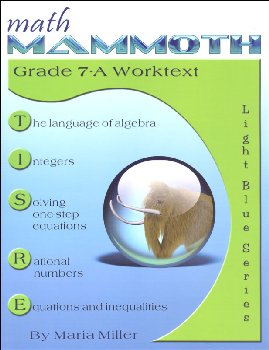# Math Mammoth Light Blue Series Grade 7-A Worktext

# BM-062261

Bargain Price: \$6.26
Retail: \$14.95
Save: 58.13% (\$8.69)
No more Bargain Versions available to purchase.Item #: BM-062261 9781954358027 7

#### Publisher Description:

The two books (worktext part A and part B) deal with

• introduction to basic algebra concepts
• integers and their operations
• solving one-step equations, including with negative numbers
• operations with negative rational numbers
• solving linear equations and writing equations for word problems
• graphing linear equations and an introduction to the concept of slope
• ratios, rates, proportions, and percent
• geometry: angle relationships, compass & ruler constructions, drawing problems, Pi and the area and circumference of a circle, cross-sections formed when cutting solids, surface area, and volume
• the Pythagorean theorem
• probability
• statistics

Math Mammoth Grade 7-A and Grade 7-B worktexts comprise a complete math curriculum for seventh grade mathematics studies. This is a pre-algebra course, and students can continue to an algebra 1 curriculum after studying it.

The curriculum meets the Common Core Standards (CCS) for grade 7 but also exceeds them in several places. For example, we study linear equations in more depth than required in grade 7 CCS, and the curriculum includes the Pythagorean Theorem, which in the CCS is in grade 8. You can access a document detailing the alignment information at this link or in the download version of this curriculum.

We start out with an introduction to basic algebra, which is in many ways a review of the same topics from 6th grade. The first chapter reviews the order of operations, the concepts of an expression and equation, and the distributive property. Students learn about the commutative and associative properties of addition and multiplication, and they simplify expressions that don't involve negative numbers.

In chapter 2, we study integers and their operations in detail. Some of this is, again, review from 6th grade, and some of it is new. The four operations of integers are explained with the help of two visual models: the number line and counters, hopefully providing an intuitive understanding of the processes. Students need to be able to add, subtract, multiply, and divide integers when they learn to solve equations in chapter 3. In the end of the chapter students also learn about negative fractions.

The lesson about distance contains a formula that may look unfamiliar to the teacher. You can find the distance between two integers by taking the absolute value of their difference. In symbols, the distance between a and b is | a − b | . The idea behind the formula is simple, though, and most people use that idea instinctively without knowing about the formula. For example, how far apart from each other are 14 and 92? To solve that mentally, we find their difference, but we take that difference in a positive sense. In other words, we do not calculate 14 − 92 = −78 and state that the distance is negative 78 units, but instead, we say the distance is 78 units because distance is always positive. The absolute value takes care of that: it turns any negative quantity into a positive one.

In the next chapter (chapter 3) students study simple one-step equations. They already know how the basics of how to solve these types of equations from 6th grade, but this time we use negative numbers in them.

Chapter 4 is titled Rational Numbers, which are simply fractions and certain decimals, so the student is already very familiar with them. The goal of the chapter is to be able to add, subtract, multiply, and divide both positive and negative fractions and decimals. We also solve simple equations involving fractions and decimals and learn about scientific notation and complex fractions.

The last chapter in part 7-A focuses on linear equations. The student learns to solve various types of linear equations and practices using those in simple word problems. We study linear inequalities but not to the same depth as linear equations. Lastly, the student graphs linear equations and is introduced to the concept of slope, which is the steepness of a line. The student will continue studying these topics with more details in an algebra 1 course.

Part B starts out with a study of ratios and proportions (chapter 6). Students study unit rates, proportions, proportional relationships and graphing, scaling geometric figures, floor plans, and maps.

The next chapter provides thorough lessons on the concept of percent. Students learn to solve a wide variety of problems involving percentages, including percentage of change, percentages of comparison, and simple interest problems.

Geometry is our focus in chapter 8. Students draw geometric figures using a a protractor and a ruler, and they also learn some basic geometric constructions. The other themes of this chapter are various angle relationships, area and the perimeter of a circle, conversions between units of area and of volume, surface area, volume, and cross-sections when solids are sliced with a plane.

Chapter 9 covers square roots, the Pythagorean Theorem, and its applications. I have included the Pythagorean Theorem in order to make the curriculum work as a pre-algebra course, and you can omit the entire chapter 9 if you are following the Common Core Standards for grade 7.

Chapter 10 is an introduction to probability. Besides learning the basic idea behind probability as the ratio of favorable events to all possible events, students compare experimental probabilities to theoretical ones in probability simulations and even design some on their own.

Lastly, in chapter 11, the curriculum covers statistical concepts. The major areas of study are random sampling and learning to compare two populations using some basic statistical measures and graphs.

Primary Subject
Mathematics
7
ISBN
9781954358027
Author
Maria Miller
Format
Paperback
Brand Name
Taina Maria Miller
Weight
1.45 (lbs.)
Dimensions
11.0" x 8.5" x 0.56"